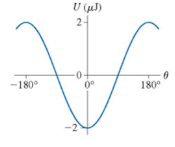# Problem: The graph shows the potential energy of an electric dipole. Consider a dipole that oscillates between ±60°What is the dipole's mechanical energy?

###### FREE Expert Solution

The potential energy of a dipole oscillating in an electric field is:

where p is the magnitude of the dipole moment, E is the field strength, and θ is the angle between the dipole moment and electric field vectors.

Total energy:

$\overline{){\mathbf{E}}{\mathbf{=}}{\mathbf{K}}{\mathbf{E}}{\mathbf{+}}{\mathbf{U}}}$

At 0°, the potential energy of the system is -2 μJ:

85% (205 ratings)###### Problem Details

The graph shows the potential energy of an electric dipole. Consider a dipole that oscillates between ±60°What is the dipole's mechanical energy?

Frequently Asked Questions

What scientific concept do you need to know in order to solve this problem?

Our tutors have indicated that to solve this problem you will need to apply the Dipole Moment concept. You can view video lessons to learn Dipole Moment. Or if you need more Dipole Moment practice, you can also practice Dipole Moment practice problems.

What professor is this problem relevant for?

Based on our data, we think this problem is relevant for Professor Jerousek's class at UCF.